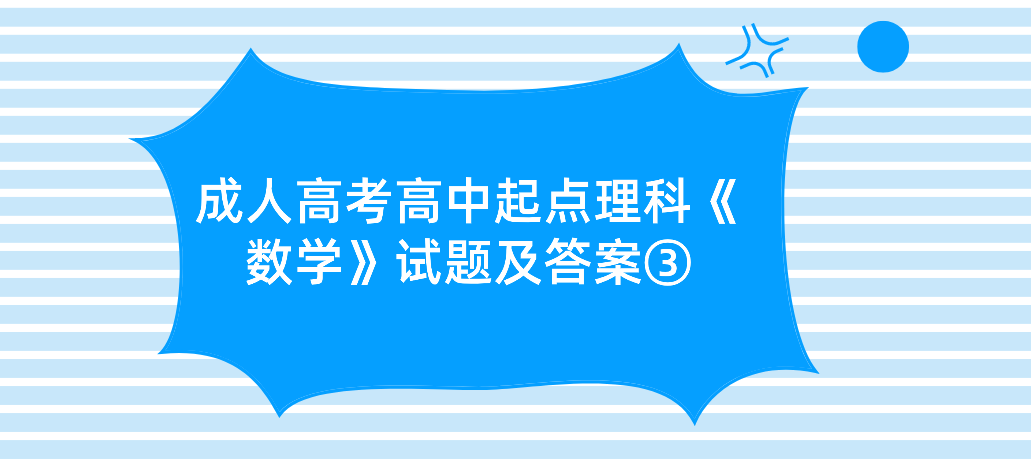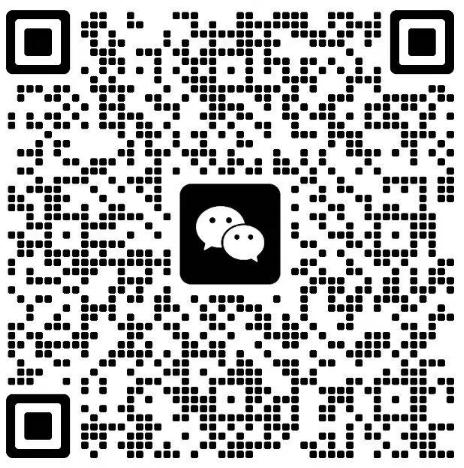# 2022年浙江成人高考高中起点理科《数学》试题及答案③11.双曲线3m-my=3的一个焦点是F(0,2),则m=()

A.1

B.1或-1

C.-1

D.2

=1,3ma2-mo2=3=>m-/x=1=4m

12、已知函数y=ax+bx+c的图像经过点(0,-1),(2,5),(-8,15),则其对称轴是()

A.x-2

B.x=-2

C.x=-1

D.x-1

c=-1

4a+2b+c=5,解得a=。b=2,c=-1,

64a-8b+c=15.1/2x+2x-1=1/2(x+2)}-3,故其对称轴为x--2.故函数的方程为y=x=-2

13.设角α的顶点在坐标原点，始边为x轴非负半轴，终边过点(-22),则sinα=()

A.V2

B.4

C.8

D.2

V2

14.三封信投入五个邮箱，不同的投法共有()

A.3种

B.5种

c.5种

D.15种

15.函数y=cos(x+/)+cosx的最大值是()

A.2

B.1

c.J2

D.0

y=cos(x+/)+cosx=-sin x+cosx=-/2sin(x-/),

16.函数y=x+1与图像交点的个数为()

A.O

B.1

C.2

D.3

[y=x+1J5-1 5+1)(-15+1 1-55),

1，得交点2

y=2

17.设甲：x-1,乙：x3-3x+2=0,则()

A.甲是乙的必要条件，但不是乙的充分条件

B.甲是乙的充分条件，但不是乙的必要条件

c.甲不是乙的充分条件，也不是乙的必要条件

D.甲是乙的充分必要条件

x=1=x-3x+2=0,但x-3x+2=0=x=1或x-2,

2023年全国成人高考还有

### 浙江成考网在线客服